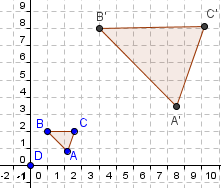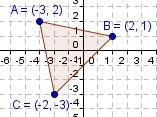Email us to get an instant 20% discount on highly effective K-12 Math & English kwizNET Programs!

#### Online Quiz (WorksheetABCD)

Questions Per Quiz = 2 4 6 8 10

### Geometry7.6 Dilation

 Dilation: A dilation is a transformation that stretches or shrinks a figure to create a similar figure. It is a type of similarity transformation. In a dilation, a figure is enlarged or reduced with respect to a fixed point called center of dilation. The scale factor of a dilation is the ratio of a side length of the image to the corresponding side length of the original figure. You can describe a dilation with respect to the origin with the notation (x,y) � (kx,ky), where k is the scale factor. If 0 < k < 1, the dilation is a reduction. If k>1, the dilation is an enlargement.Directions: Using graph paper and dilate the following figures using scale factor of 1, 2, 3, 4 and 1/2.Answer the following questions. Also write at least 10 examples of your own.
 Q 1: Draw a dilation of the polygon with the given vertices: A(0,0), B(0, 3), C(2, 4), D(2, -1) using the scale factor of 13/2. The coordinates of the vertex D of the image is ______. (13, 13/2)(-13/2, 13)(13, -13/2) Q 2: Draw a dilation of the polygon with the given vertices: A(-2,1), B(-4, 1), C(-2, 4) using the scale factor of 3. The coordinates of the vertex C of the image is ______. (-6, 3)(-6, 12)(6, 12) Q 3: A triangle has a vertices A(4, -4), B(8, 2) and C(8, -4). Draw the image (Triangle DEF) of the original triangle ABC after a dilation with a scale factor of 1/2. The coordinates of point C of the image are ________.(4, 1)(1, -1)(4, -2) Q 4: What is the scale factor of the dilated figure.Answer: Question 5: This question is available to subscribers only! Question 6: This question is available to subscribers only!